# The TRANSREG Procedure

### Centering

You can use transformation options to center and standardize the variables in several ways. For example, the following MODEL statement creates three independent variables, x,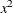, and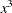:

model identity(y) = pspline(x);


The variables are not centered.

When the CENTER t-option is specified, as in the following statement, the independent variable is centered before squaring and cubing:

model identity(y) = pspline(x / center);


The three independent variables are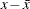,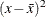, and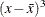.

Since operations such as squaring occur after the centering, the resulting variables are not always centered. The CENTER t-option is particularly useful with polynomials since centering before squaring and cubing can help reduce collinearity and numerical problems. For example, if one of your variables is year, with values all greater than 1900, squaring and cubing without centering first will create variables that are all essentially perfectly correlated.

When the TSTANDARD= CENTER t-option is specified, as in the following model, the three independent variables are squared and cubed and then centered:

model identity(y) = pspline(x / tstandard=center);


The three independent variables are,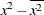, and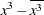.

You can specify both the CENTER and TSTANDARD=CENTER t-options to center the variables, then square and cube them, and then center the results, as in the following statement:

model identity(y) = pspline(x / center tstandard=center);


The three independent variables are,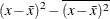, and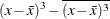.